ROV21_6.MWS

Chapter 21. Some Other Classes of Surfaces

> restart:

21.1 Canal Surfaces and Tubes

The command tubeplot allows one to plot a tube with an arbitrary space curve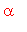(t) of centers (see Section 8.1.1).

A program for a canal surface over an arbitrary curve:

> with(linalg):

```Warning, protected name norm has been changed and unprotected
```

```Warning, protected name trace has been changed and unprotected
```

> x:=u -> u: y:=u -> u^2: z:=u -> u/2: R:=u->u:

> r:=array([x(u), y(u), z(u)]); # define yours functions x(u),y(u),z(u)> R:=R(u); u0:=0; u1:=2; # define function R(u)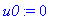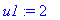> dr:=map(diff, r, u); ddr:=map(diff, r, u\$2);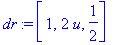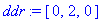> tau:=scalarmul(dr, 1/norm(dr, 2)); b:=crossprod(dr, ddr);> beta:=scalarmul(b, 1/simplify(norm(b, 2)));> nu:=crossprod(beta, tau);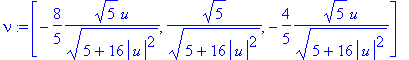> rr:=evalm(r+scalarmul(nu, R*cos(v))+scalarmul(beta, R*sin(v)));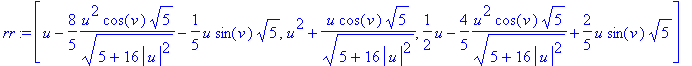> plot3d(rr, u=u0..u1, v=0..2*Pi, axes=none);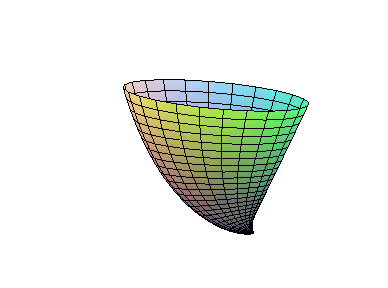Exercises .

1. Write down equations of the canal surface with R(u)=ku and the tube over the circular and conic helix,

plot surfaces ( Sea shell ).

2. Plot the tube over the torus knot using the following program:

> N:=10: t_tub:=plots[tubeplot]({[10*cos(t), 10*sin(t), 0, t=0..2*Pi, radius=2, numpoints=10*N, tubepoints=2*N],[cos(t)*(10+4*sin(9*t)), sin(t)*(10+4*sin(9*t)), 4*cos(9*t), t=0..2*Pi, radius=1, numpoints=trunc(37.5*N), tubepoints=N]}, scaling=constrained): t_tub;3. Plot the canal surface defined by moving the circle of radius 4+sin(2t) along the circle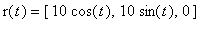of radius 10

> plot3d([(12+(4+sin(4*v))*sin(u))*cos(v), (12+(4+sin(4*v))*sin(u))*sin(v), (4+sin(4*v))*cos(u)], u=0..2*Pi, v=0..2*Pi, grid=[20,60], scaling=constrained);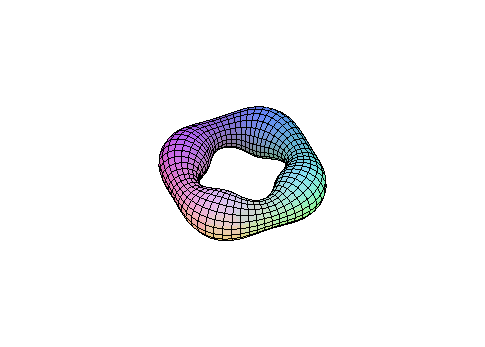4. Plot eight different figure eights .

Solution . The {Lissajous curve}for n=2 has the shape of a figure eight.

We plot the surface of revolution of the figure eight

> plot3d([sin(u)*sin(v), cos(u)*sin(u)*sin(v), cos(v)*sin(v)], v=0..2*Pi, u=0..2*Pi, grid=[40,50],scaling=constrained);We plot the surface defined by moving of an figure eight along another figure eight.

> plot3d([cos(u)*sin(2*v),sin(u)*sin(2*v),sin(v)],v=0..2*Pi, u=0..2*Pi, grid=[40,50], scaling=constrained);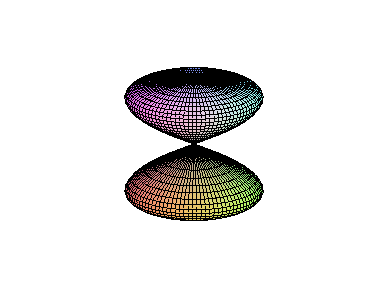>

Let us plot also the tube over an eight.

21.2 Translation Surfaces

Exercises .

1. Prove that the following surfaces are translation surfaces:

(a) The elliptical and hyperbolic paraboloids.

(b) The {Bohemian dome} (translation of the ellipse along the circle).

> r:= [8*cos(u), 8*sin(u)+3*cos(v), 3*sin(v)];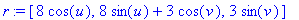> plot3d(r, v=0..-Pi, u=0..2*Pi, scaling=constrained);>

21.3 Twisted Surfaces

Exercise . Plot the Lissajous curve, |u|<=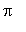, with n= 4, and its twisted surface.

> plot([sin(4*t), sin(t), t=0..2*Pi],scaling=constrained); # the curve> plot3d([(2+cos(u/2)*sin(4*v)-sin(u/2)*sin(v))*cos(u), (2+cos(u/2)*sin(4*v)-sin(u/2)*sin(v))*sin(u), sin(u/2)*sin(4*v)+cos(u/2)*sin(v)], u=-Pi/8..3*Pi/2, v=0..2*Pi, grid=[30,80],scaling=constrained); #the surface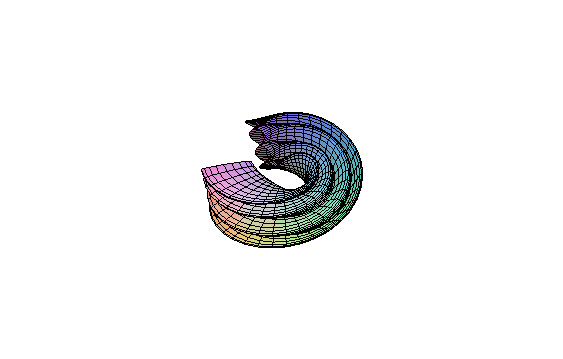>

21.4 Parallel Surfaces (Equidistants)

Exercises .

3. Plot parallel surfaces for an ellipsoid; the hyperboloid of one sheet; the catenoid; the Mobius strip.

Solution . We plot some parallel surfaces for (part of) a ellipsoid:

> restart: with(linalg): r:=[cos(u)*cos(v), cos(u)*sin(v),2*sin(u)]:

```Warning, protected name norm has been changed and unprotected
```

```Warning, protected name trace has been changed and unprotected
```

> ru:=map(diff,r,u): rv:=map(diff,r,v):

> nn:=crossprod(ru, rv): n:=scalarmul(nn, 1/norm(nn,2)):

> p1:=plot3d(evalm(r+scalarmul(n,-1.5)), u=-Pi/3..Pi/3,v=Pi/4..2*Pi): p2:=plot3d(evalm(r+scalarmul(n,-1)), u=-Pi/3..Pi/3,v=Pi/4..2*Pi): p3:=plot3d(evalm(r+scalarmul(n,-0.6)), u=-Pi/3..Pi/3,v=Pi/4..2*Pi): p4:=plot3d(evalm(r+scalarmul(n,0.2)), u=-Pi/3..Pi/3,v=Pi/4..2*Pi):

> plots[display3d]({p1, p2, p3, p4});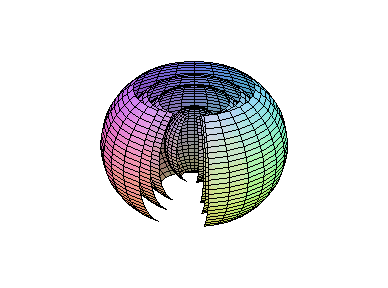To plot parallel surfaces for the hyperboloid of one sheet we set in the above program

> r:=[cosh(u)*cos(v), cosh(u)*sin(v), sinh(u)]: ru:=map(diff,r,u): rv:=map(diff,r,v):nn:=crossprod(ru, rv): n:=scalarmul(nn, 1/norm(nn,2)): nn:=crossprod(ru, rv): n:=scalarmul(nn, 1/norm(nn,2)): p1:=plot3d(evalm(r+scalarmul(n,-1.5)), u=-Pi/3..Pi/3,v=Pi/4..2*Pi): p2:=plot3d(evalm(r+scalarmul(n,-1)), u=-Pi/3..Pi/3,v=Pi/4..2*Pi): p3:=plot3d(evalm(r+scalarmul(n,-0.6)), u=-Pi/3..Pi/3,v=Pi/4..2*Pi): p4:=plot3d(evalm(r+scalarmul(n,0.2)), u=-Pi/3..Pi/3,v=Pi/4..2*Pi): plots[display3d]({p1, p2, p3, p4});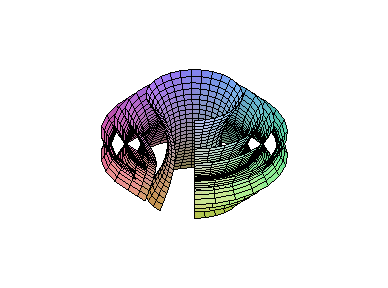>

Note that a parallel surface for the Mobius strip has two sides (is orientable).

> r:=[(5+cos(v/2)*u)*cos(v), (5+cos(v/2)*u)*sin(v), sin(v/2)*u];> ru:=map(diff,r,u): rv:=map(diff,r,v):

> nn:=crossprod(ru, rv): n:=scalarmul(nn, 1/norm(nn,2)):

> p1:=plot3d(r, u=-1..1, v=0..2*Pi, grid=[10,50]): p2:=plot3d(evalm(r+scalarmul(n,1)), u=-1..1, v=0..4*Pi, grid=[10, 80]):

> plots[display3d]({p1, p2});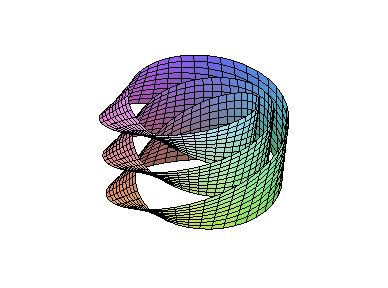>

21.5 Pedal and Podoid Surfaces

Exercises .

2. Plot the pedal surfaces of the ellipsoid with respect to its center, one of its vertices, and any external point.

> restart: with(linalg): r:=([cos(u)*cos(v), 2*cos(u)*sin(v), 3*sin(u)]);

```Warning, protected name norm has been changed and unprotected
```

```Warning, protected name trace has been changed and unprotected
```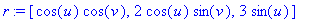> ru:=map(diff,r,u): rv:=map(diff,r,v):

> nn:=crossprod(ru, rv): p:=array([3,0,0]):

> coef:=simplify(dotprod(evalm(r-p), nn,orthogonal)/dotprod(nn, nn,orthogonal));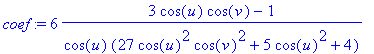> rr:=evalm(p+scalarmul(nn, coef)):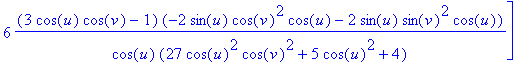> plot3d(rr, u=-Pi/2..Pi/2, v=Pi/2..2*Pi,grid=[25,30]);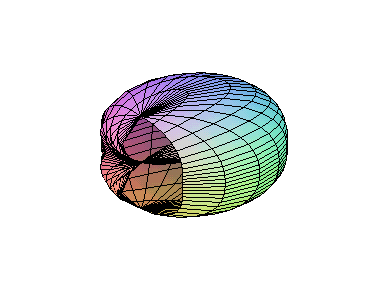>

21.6 Cissoidal and Conchoidal Maps

Since implicitplot3d gives a coarse image, we use the parametric equations of part of the cissoid

x= t, z=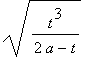(0<= t< 2a) and plot the surface of revolution, by formulas (20.1a)

r= [ u,,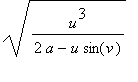] (0<= u < 2a, 0<= v< 2).

> plot(sqrt(x^3/(2-x)), x=-1.8..1.8); # a=1> plot3d([u,sqrt(u^3/(2-u))*cos(v), sqrt(u^3/(2-u))*sin(v)], u=0..1.9, v=0..2*Pi);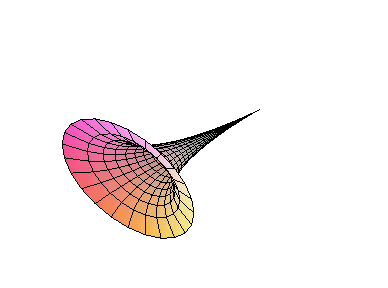2. Write down the equation and plot the {conchoidal surface of the plane} {z=a} with l=1.

> plots[polarplot]([2/cos(t)-1, t, t=-1.5..1.5]); # profile curve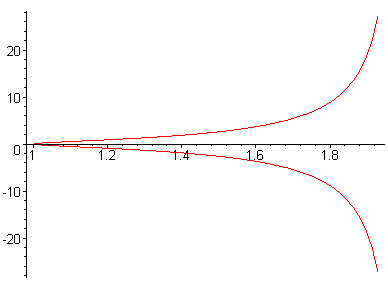> plot3d([(2/sin(u)-1)*cos(u)*cos(v), (2/sin(u)-1)*cos(u)*sin(v), (2/sin(u)-1)*sin(u)], u=0..0.8, v=0..2*Pi); # surface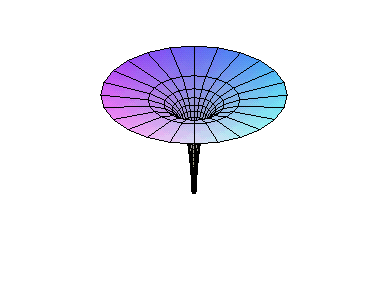>

21.7 Inversion of a Surface

Example 1 .

1a. Inversion with center O and radius 1 of the circular helix,is the curve.

> restart: with(linalg): r:=[3+cos(u), sin(u), 0.1*u];

```Warning, protected name norm has been changed and unprotected
```

```Warning, protected name trace has been changed and unprotected
```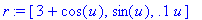> ri:=(scalarmul(r, 1/norm(r, 2)^2)):

> plots[spacecurve](r, u=0..8*Pi, numpoints=200,axes=framed);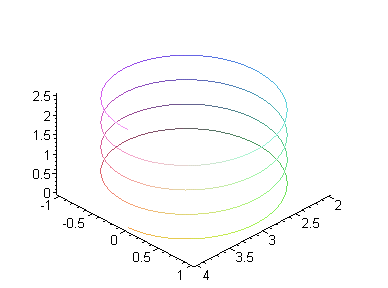> plots[spacecurve](ri, u=-25*Pi..25*Pi, numpoints=999, axes=boxed);1b. The command inversion(Q, P, S) from the library geom3d plots the inversion (the object Q ) of a point, plane,

or sphere P with respect to the sphere S .

> restart: with(geom3d):

```Warning, existing definition for polar has been overwritten
```

> point(A, 1,2,-1): point(B, 0,0,-1): point(C, 3,0,0):

> plane(P, [A,B,C]): sphere(S, [point(O,0,0,0),1]):

> inversion(F,P,S):

> draw([S, F, P(style=patchnogrid,color=maroon)], style=wireframe, view=[-1..1,-1..1,-2..1], title=`inversion of a plane with respect to a sphere`);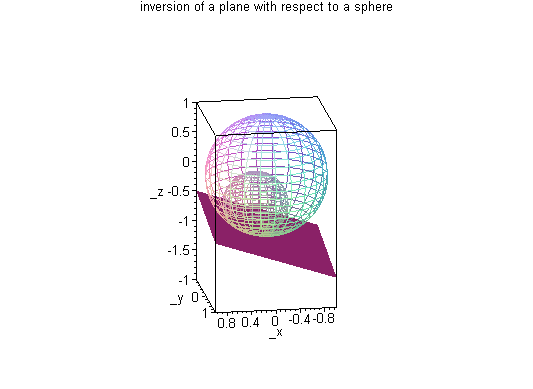>

Let us plot an inversion image of a paraboloid of revolution; the hyperboloid of one sheet; and the inversion images

of their profiles:

> restart: with(linalg): r:=[u*cos(v), u*sin(v), u^2+1]:

```Warning, protected name norm has been changed and unprotected
```

```Warning, protected name trace has been changed and unprotected
```

> ri:=(scalarmul(r, 1/norm(r, 2)^2)):

> plot3d(r, u=1..7, v=-Pi..(3/4)*Pi); # paraboloid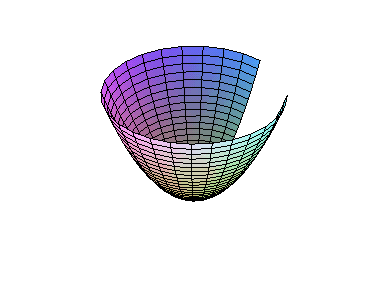> plot3d(ri, u=1..7, v=-Pi..Pi); # inversion of paraboloid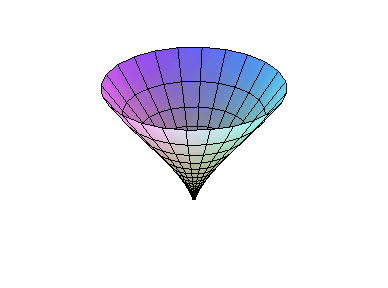> r:=[sinh(u)*cos(v), sinh(u)*sin(v), cosh(u)+1]:

> ri:=scalarmul(r, 1/norm(r,2)^2): # hyperboloid

> plot3d(ri, u=1..7, v=-Pi..Pi); # inversion of hyperboloid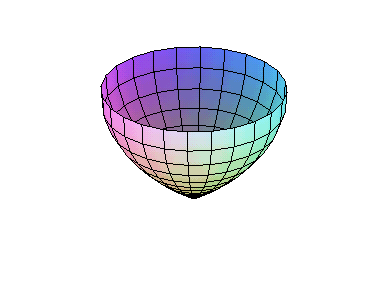> r2:=[u, u^2+1]: # parabola

> r2:=[sinh(u), cosh(u)+1]: # hyperbola

> r2i:=scalarmul(r2, 1/norm(r2, 2)^2):

> plot([r2, r2, u=-2..2], scaling=constrained); # curve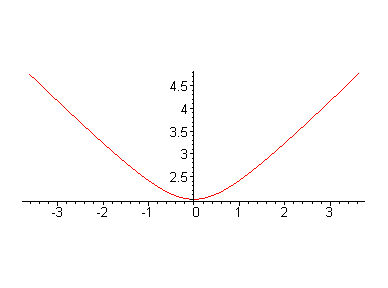> plot([r2i, r2i, u=-6..6], scaling=constrained); # inversion of curve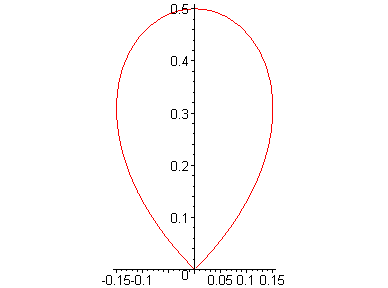Exercise . Write down the equation and plot some cyclides of Dupin.

Hints . We plot two images, of the torusunder inversion with center O and radius 1: when a=2, b=8, and when a=1, b=4.

> r:=[8+(2+cos(u))*cos(v), (2+cos(u))*sin(v), sin(u)]:

> r:=[4+(1+cos(u))*cos(v), (1+cos(u))*sin(v), sin(u)]:

> invr:=scalarmul(r, 1/(norm(r, 2)^2)):

> plot3d(invr, u=-Pi..Pi, v=-Pi..Pi, grid=[40,60], scaling=constrained);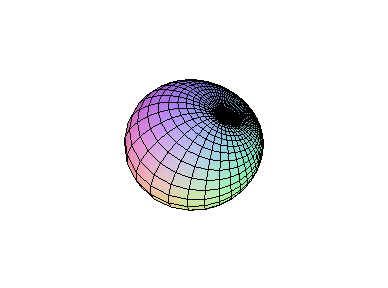>